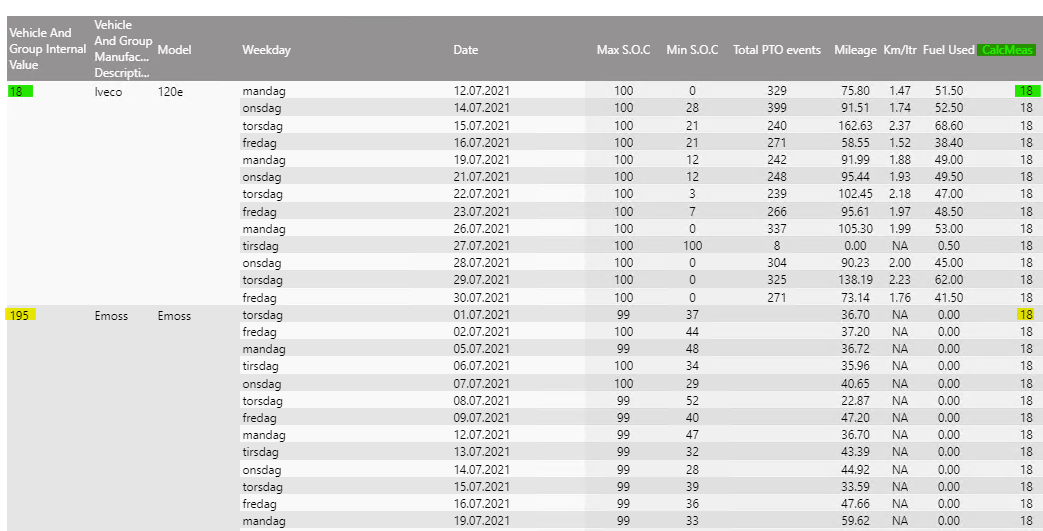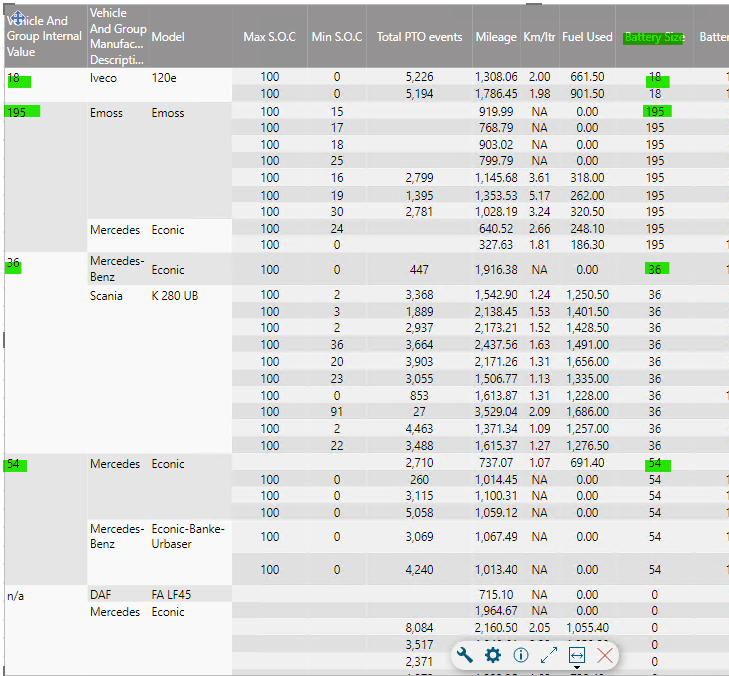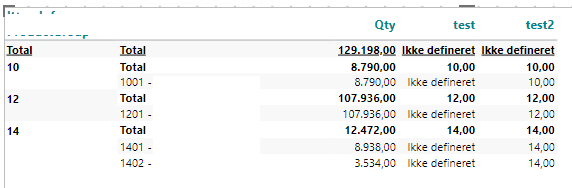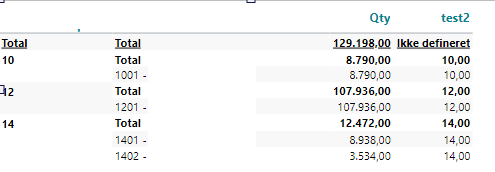# Calculation is returning only the first dimension member value

Hi,

I'm struggling with a calculation that would enable me to use the dimension "value" for further calculations.

At this stage I'm able to get the first dimension member as a calculated member, but the values remains the same regardless the dimension member value. For dimension member 195 the CalcMeas should return 195 rather then 18.The calculation is as following:

if allcount(@"[UD18]", 0, m1, 0) > -1 then 18 else if allcount(@"[UD195]", 0, m1, 0) > -1 then 195 else if allcount(@"[UD36]", 0, m1, 0) > -1 then 36 else 0.

Does anyone has a suggestion how the above can be achieved?

Br.

Steffi

• Shouldn't your @[UD18]" be after the first ","? As the syntax is (column, row, measure).

What if you type something like:

if allcount(0, d1:0, m1) = allcount(0, d1:@"[UD18]", m1) then 18 else if allcount(0, d1:0, m1) = allcount(0, d1:@"[UD195]", m1) then 195 else if allcount(0, d1:0, m1) = allcount(0, d1:@"[UD36]", m1) then 36 else 0.

• Maybe this will only give you the right numbers in the first row for each value?? Unfortunately I haven't got time to make a test.. But maybe it could help you a step further..

• Hi Louise,

that unfortunately doesn't do the trick, it returns the CalcMeas as undefined.

• But why do you type @"[UD18]" and not just @"" etc.? In the first column in your screendump I can only see 18, 195 and not "UD18"..

• Maybe you should try to split it in steps.

One new column with allcount(0, d1:0, m1)

One new column with allcount(0, d1:0@", m1)

etc.

To see if you can reach the right values in your equation?

• As far I recall there has been an issue doing it that way and UD should have been added. But even when I remove the UD from my first calculation, it remains returning just the first dimension member value.

• You should be able to refer to allcount(0,@"";"[Iveco]";"[120e]";"[mandag]";"[12.07.2021]",0,0) , but I don't know if you can just refer to the first column? I hope that others have a solution...

• The allcount calculation has been returning some strange values and didn't work at all for me. But I was able to find a workaround that solves my issues.

When a dimension member changes (1,2,3,4) I insert the desired value and hide afterword's the dimension member, to avoid duplicates. It is not a dynamic solution, but it works for my purposes.

if allcount(d1, d1:0(l(1,0,0,0,0)), m1) = 1 then 18 else if allcount(d1, d1:0(l(1,0,0,0,0)), m1) = 2 then 195 else if allcount(d1, d1:0(l(1,0,0,0,0)), m1) = 3 then 36 else if allcount(d1, d1:0(l(1,0,0,0,0)), m1) = 4 then 54 else 0• Glad to hear that you found a solution. Thanks a lot for sharing! :-)

• Hi Steppi, I know you found a solution, but maybe this could work, an be easier to alter later if needed?I didn't have any samples where I could make 4 dimensions, so in my case its only 2, but the same logic applies in your case, with a small alteration in the syntax.

`if sum(d1, 0(l(1,0)), 0) = sum(d1, @"", m1) then 10 else if sum(d1, 0(l(1,0)), 0) = sum(d1, @"", m1) then 12 else if sum(d1, 0(l(1,0)), 0) = sum(d1, @"", m1) then 14 else 0`

And then second column:

`if allcount(d1, all(c), m1) > 0 then sum(c1, 0, 0) else sum(c1, 0(l(1,0)), 0)`

In your case you change all the places where I have

0(l(1,0))

to

0(l(1,0,0,0)) if you have 4 dimension levels as in your first example.

And then you just hide the first column